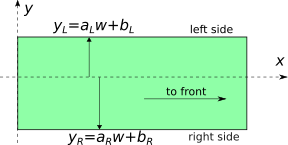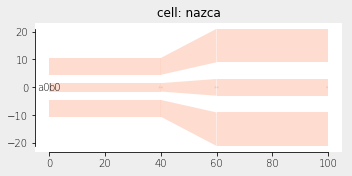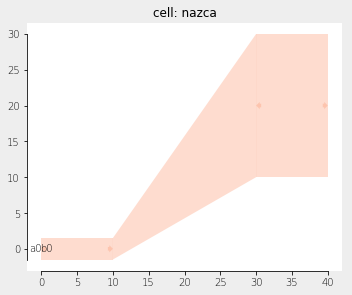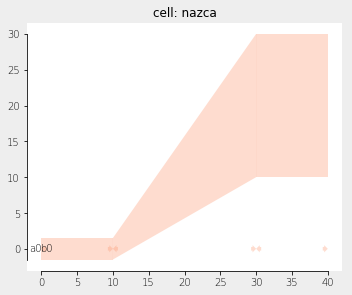Home Forums Nazca Connecting two dissimilar ribbons

Viewing 5 posts - 1 through 5 (of 5 total)
• Author
Posts
• #5707

Hey,

I’m currently working on the design of a PCB in Nazca and I have the situation that I have two ribbons with a pitch of 150 um, a width of 75 um and 30 traces close to the chip and I want to connect those to one ribbon with a pitch of 500 um, a width of 300 um and 60 traces. My question is twofold:

How do I add ribbons to my design? I’ve seen it demonstrated once, but I’ve forgot the syntax.

Is it currently possible to connect two ribbons that aren’t equal or is there a smart way to create that possibility?

#5711

Dear mjbvrijn,

As of Nazca 0.5.4 a waveguide in a specific layer inside a xsection can be defined by providing the left edge and right edge of the waveguide independently. Multiple of such guides can be defined inside a single xsection. The edge is define by y = a*w+b, as clarified in the picture below. Parameter w is the width setting of the xsection.As long as you can describe an edge of by a single y = a*w +b statement, a standard inteconnect taper will work for a case as you describe, as shown in the next example. Note that a and b parameters for an edge are provided as tuples (a, b) via the keywords leftedge and rightedge:

``````import nazca as nd

nd.add_layer2xsection(xsection='GSG', leftedge=(0.5, 0), rightedge=(-0.5, 0), layer='L1')
nd.add_layer2xsection(xsection='GSG', leftedge=(3.5, 0), rightedge=(1.5, 0), layer='L1')

tr.strt(length=40).put(0)
tr.taper(length=20, width2=6.0).put()
tr.strt(length=40, width=6.0).put()

nd.export_plt()``````If you start with the dimension on the left (y1) and right (y2) side of the taper in the above example you can find a and b of an edge as follows:

y1 = a1 * w1 + b1

y2 = a2 * w2 + b2

and solve for y1 =  y2

This gives

a = (y1 – y2) / (w1 – w2)

b = (y2*w1 – y1*w2) / (w1 – w2)

and note that the widths w1 and w2 can be considered scaling numbers instead of actual widths of a guide in the xsection.

Ronald

#5712

Dear Ronald,

As I understand it you are proposing to use the definition of the xsection to create a large ribbon that can have nicely defined taper. I’ve tried to use this, but I don’t think you can change the pitch of the ribbon in this way and it’s a bit challenging to connect this to the rest of the circuit.

``````import nazca as nd

n_ribbon=20
for i in range(0,n_ribbon):
nd.add_layer2xsection(xsection='GSG', leftedge=(1.5-n_ribbon+2*i, 0), rightedge=(0.5-n_ribbon+2*i, 0), layer='L1')

tr.strt(length=40).put(0)
tr.taper(length=20, width2=6.0).put()
tr.strt(length=40, width=6.0).put()

nd.export_plt()``````

At the moment I’m using a slightly scruffy method that only works because I’m working in metals that only use 1 layer, where I use a polygon to define a taper based on the pin information.

``````import nazca as nd

nd.add_layer2xsection(xsection='metal_strt', leftedge=(0.5, 0), rightedge=(-0.5, 0), layer='L1')

in_wg=ic.strt(10).put(0,0)
out_wg=ic.strt(length=10,width=20.0).put(30,20)

width_in=in_wg.pin['b0'].width
x_in=in_wg.pin['b0'].xya()
y_in=in_wg.pin['b0'].xya()

width_out=out_wg.pin['a0'].width
x_out=out_wg.pin['a0'].xya()
y_out=out_wg.pin['a0'].xya()

nd.Polygon(layer=1,points=[(x_in,y_in+width_in/2),(x_in,y_in-width_in/2),(x_out,y_out-width_out/2),(x_out,y_out+width_out/2)]).put(0,0)

nd.export_gds()``````

For now this works. If you were suggesting something else and I misinterpreted your code, I’d be happy with a cleaner solution.

Kind regards

#5714

Dear mbjvrijn,

The shape in your second code samplecan be reproduced using the relations for a and b in my previous post. In the code below I took y1 and y2 from the output of your code. The centre line of the interconnect lies at y=0.

``````import nazca as nd

# get (a, b) for the left and right edge:
def get_edge(y1, y2):
w1 = 1.0 # w1 and w2 can be chosen arbitrary, but not the same
w2 = 2.0
a = (y1 - y2) / (w1 - w2)
b = (y2*w1 - y1*w2) / (w1 - w2)
return a, b

al, bl = get_edge(y1=1.5, y2=30)
ar, br = get_edge(y1=-1.5, y2=10)

# The actual xsection definition:

nd.add_layer2xsection(xsection='metal_strt', leftedge=(al, bl), rightedge=(ar, br), layer='L1')
ic = nd.interconnects.Interconnect(xs='metal_strt', width=1.0, radius=40) # reuse w1 value from (a, b) calc.

ic.strt(10).put(0)
ic.taper(20, width2=2.0).put() # reuse w2 value from the (a, b) calculation
ic.strt(10, width=2.0).put()

nd.export_plt()``````Ronald

#5825

Dear mbjvrjn,

A tutorial on this topic has been added in xsections and layers

Ronald

Viewing 5 posts - 1 through 5 (of 5 total)
• You must be logged in to reply to this topic.